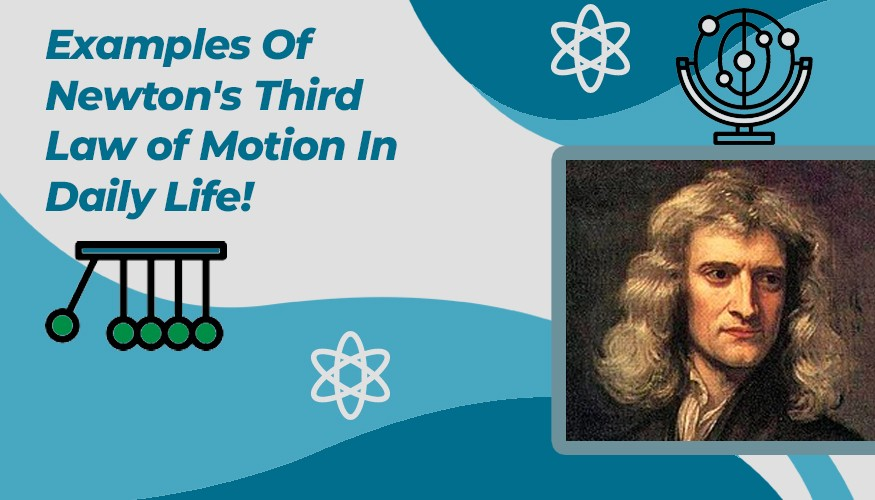# Newton?s Third Law: Everyday Life Explained By An Equal And Opposite Force!785Playing hockey, driving a car, and even simply taking a walk are all everyday examples of Newton's laws of motion. Compiled in 1687 by English mathematician Isaac Newton, the three main laws describe forces and motion for objects on Earth and throughout the universe.

Newton's laws of motion are three physical laws that, together, laid the foundation for classical mechanics. They describe the relationship between a body and the forces acting upon it, and its motion in response to those forces

## Newtons Third Law:

Formally stated, Newton's third law is: For every action, there is an equal and opposite reaction.

The statement means that in every interaction, there is a pair of forces acting on the two interacting objects. The size of the forces on the first object equals the size of the force on the second object.

### Application of Newton?s Third Law:

Examples of Newton's third law of motion are ubiquitous in everyday life. For example, when you jump, your legs apply a force to the ground, and the ground applies an equal and opposite reaction force that propels you into the air.

Engineers apply Newton's third law when designing rockets and other projectile devices

### Conclusion:

Newton's law is very important because they tie into almost everything we see in everyday life. These laws tell us exactly how things move or sit still, like why you don't float out of bed or fall through the floor of your house.

### Researcher Found!

Everyday studies are conducted and designing is done using Newton?s laws in NASA